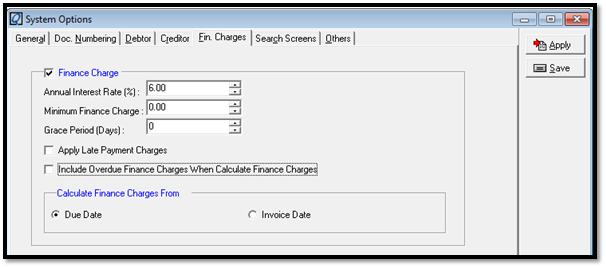Finance Charges Calculation Formula – PART 1

Settings

• Tick “Finance Charge”
• Insert Number for Annual Interest Rate (%)
• Select “Due Date”Calculation for January

= [(Jan Invoice Amount   x Annual Interest Rate)  x (Total Day of Jan – Due Date)]  / 365

Calculation for February

= [(Feb Invoice Amount    x Annual Interest Rate)  x (Total Day of Feb – Due Date)]  / 365

+ [(Jan Invoice Amount     x Annual Interest Rate)  x Total Day of Feb]                         / 365

Calculation for March

= [(Mar Invoice Amount  x Annual Interest Rate)  x (Total Day of Mar – Due Date)]  / 365

+ [(Feb Invoice Amount   x Annual Interest Rate)  x Total Day of Mar]                       / 365

+ [(Jan Invoice Amount   x Annual Interest Rate)  x Total Day of Mar]                        / 365

Calculation for April

= [(Apr Invoice Amount  x Annual Interest Rate)  x (Total Day of Apr – Due Date)]  / 365

+ [(Mar Invoice Amount  x Annual Interest Rate)  x Total Day of Apr]                     / 365

+ [(Feb Invoice Amount   x Annual Interest Rate)  x Total Day of Apr]                     / 365

+ [(Jan Invoice Amount   x Annual Interest Rate)  x Total Day of Apr]                     / 365

Finance Charges Calculation Formula – PART 2

Settings

• Tick “Finance Charge”
• Insert Number for Annual Interest Rate (%)
• Tick “Include Overdue Finance Charges When Calculate Finance Charges”
• Select “Due Date”
• Set 10 Days Term (Set at Debtor or Invoice)

Calculation for January

= [(Jan Invoice Amount   x Annual Interest Rate)  x (Total Day of Jan – Due Date - Term)]   / 365

Calculation for February

= [(Feb Invoice Amount   x Annual Interest Rate)  x (Total Day of Feb – Due Date - Term)]  / 365

+ [(Jan Invoice Amount   x Annual Interest Rate)  x Total Day of Feb]                                 / 365

+ [(Jan Finance Charges   x Annual Interest Rate)  x (Total Day of Feb - Term)]                   / 365

Calculation for March

= [(Mar Invoice Amount  x Annual Interest Rate)  x (Total Day of Mar – Due Date - Term)]  / 365

+ [(Feb Invoice Amount   x Annual Interest Rate)  x Total Day of Mar]                                / 365

+ [(Jan Invoice Amount   x Annual Interest Rate)  x Total Day of Mar]                                / 365

+ [(Feb Finance Charges  x Annual Interest Rate)  x (Total Day of Mar - Term)]                  / 365

+ [(Jan Finance Charges   x Annual Interest Rate)  x Total Day of Mar]                                / 365

Calculation for April

= [(Apr Invoice Amount  x Annual Interest Rate)  x (Total Day of Apr – Due Date - Term)]  / 365

= [(Mar Invoice Amount  x Annual Interest Rate)  x Total Day of Apr]                                 / 365

+ [(Feb Invoice Amount   x Annual Interest Rate)  x Total Day of Apr]                                 / 365

+ [(Jan Invoice Amount   x Annual Interest Rate)  x Total Day of Apr]                                 / 365

+ [(Mar Finance Charges  x Annual Interest Rate)  x (Total Day of Apr - Term)]                   / 365

+ [(Feb Finance Charges  x Annual Interest Rate)  x Total Day of Apr]                                 / 365.

+ [(Jan Finance Charges x Annual Interest Rate) x Total Day of Apr]                                    / 365